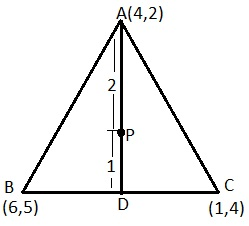Newbie

# Let A (4, 2), B (6, 5) and C (1, 4) be the vertices of ∆ ABC. (i) The median from A meets BC at D. Find the coordinates of point D. Q.7(1)

• 0

How i solve the ncert class 10 question  of coordinate geometry  in a easy way . Please give me the best solution of the coordinate geometry  of exercise 7.4 question no. 7(1) problems with simple  language .Let A (4, 2), B (6, 5) and C (1, 4) be the vertices of ∆ ABC. (i) The median from A meets BC at D. Find the coordinates of point D.

Share

1.